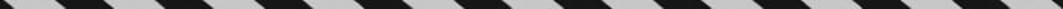﻿ ﻿COMPLEX FRACTIONS - Fractions, Decimals, and Percents - TOPICS IN ARITHMETIC - SAT SUBJECT TEST MATH LEVEL 1

## TOPICS IN ARITHMETIC## CHAPTER 3Fractions, Decimals, and Percents### COMPLEX FRACTIONS

A complex fraction is a fraction, such as, that has a fraction in its numerator, denominator, or both.

Key Fact B10

A complex fraction can be simplified in two ways: (i) multiply every term in the numerator and denominator by the least common multiple of all the denominators that appear in the fraction or (ii) simplify the numerator and the denominator and then divide.

EXAMPLE 10: To simplify, multiply each term by 12, the 2 34 LCM of 6 and 4:or write﻿